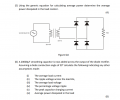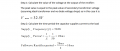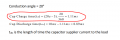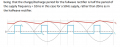# To calculate the Capacitor charge time in this circuit why do we use the supply period instead of the period output by the rectifier?

#### Mojo Pin__

Joined Apr 13, 2019
83
Hello,

I am working through this tutorial question 6:I understand how to approach the question but when I was going through the solutions I don't understand why the professor chooses the supply period to calculate the Capacitor charge time. Surely the capacitor just sees a rectified wave with period of 10ms.

The way he explains it is this:I thought it would be:

Cap charge time = (10 x 10^-3) x 20/360 = 0.56ms
Cap discharge time = (10 x 10^-3) x 340/360 = 9.44ms

But when I run a simulation it does appear to be approximately 1.11ms

I'm confused about why the charge time doesn't change because the way I look at it is that the capacitor is discharging for less time so surely it wouldn't have to charge up as much?

#### MrChips

Joined Oct 2, 2009
27,679
Cycle period of 10ms and conduction angle are two different things.
The capacitor does not receive charge during the full 10ms.
The capacitor receives charge only when the rectifiers are forward bias. This is the purpose of conduction angle.
Determining the conduction angle and the shape of the current pulse is a messy calculation. It is also dependent on both the values of the capacitance and the load. Using a value is 20° is arbitrary and simply used for an exercise only.

•Mojo Pin__

#### Mojo Pin__

Joined Apr 13, 2019
83
Cycle period of 10ms and conduction angle are two different things.
The capacitor does not receive charge during the full 10ms.
The capacitor receives charge only when the rectifiers are forward bias. This is the purpose of conduction angle.
Determining the conduction angle and the shape of the current pulse is a messy calculation. It is also dependent on both the values of the capacitance and the load. Using a value is 20° is arbitrary and simply used for an exercise only.
Hi, thank you for your response!

What you have said makes sense, but what is the reason we would use 20ms instead of 10ms when calculating the capacitor charge time? In the solutions to this question the professor starts by saying:So as he says, if the supply frequency is 20ms and the charge/discharge period for the fullwave is half the period of the supply frequency (which would then be 10ms) then why would the capacitor charge time be:

20ms x 20°/360°
10ms x 20°/360°

I have it as:
Cap charge time = (10ms) x 20°/360° = 0.56ms
Cap discharge time = (10ms) x 340°/360° = 9.44ms

but the professor has:
Cap charge time = (20ms) x 20°/360° = 1.11ms
Cap discharge time = 10ms - 1.11ms = 8.89ms

I do think that I have this wrong I just don't understand why I am wrong,

Last edited by a moderator:

#### MrChips

Joined Oct 2, 2009
27,679
Line frequency is 50Hz.
Fullwave rectified frequency is 100Hz.

•Mojo Pin__

#### Mojo Pin__

Joined Apr 13, 2019
83
Line frequency is 50Hz.
Fullwave rectified frequency is 100Hz.
ah ok, yeah I missed that, thank you.

So if the rectified frequency is 100Hz the rectified period is 10ms which is what I thought.

So if I use this to calculate the capacitor charge time would it be:

Cap charge time = (10ms) x 20/360 = 0.56ms
Cap discharge time = (10ms) x 340/360 = 9.44ms

because when I run a simulation of this circuit it seems to give charge time closer to 1.11ms and discharge time around 8.89ms, so even though prof made a mistake he seems to have somehow got the right answer?

Apologies if I'm missing something obvious, I really appreciate your help

#### Mojo Pin__

Joined Apr 13, 2019
83
ah ok, yeah I missed that, thank you.

So if the rectified frequency is 100Hz the rectified period is 10ms which is what I thought.

So if I use this to calculate the capacitor charge time would it be:

Cap charge time = (10ms) x 20/360 = 0.56ms
Cap discharge time = (10ms) x 340/360 = 9.44ms

because when I run a simulation of this circuit it seems to give charge time closer to 1.11ms and discharge time around 8.89ms, so even though prof made a mistake he seems to have somehow got the right answer?

Apologies if I'm missing something obvious, I really appreciate your help
OH is it because it should be 10ms x 20/180 instead of 10ms x 20/360?

#### MrChips

Joined Oct 2, 2009
27,679
Yes, it is 20/180 for full wave.

However, if you are using a simulator, then it would calculate the conduction angle which might not be 20°.

•Mojo Pin__

#### MrAl

Joined Jun 17, 2014
9,633
The thing is, you have to know what that 20 degrees refers to either the full input wave cycle or the output wave cycle. For many problems like this they go by the reference cycle which is 50Hz not 100Hz that is why i believe he used 20/360 because that is the total conduction angle not just for 1/2 of the input cycle.
Other problems are like this too and the reason is usually because it does not make any sense to refer to the output when the input is the reference. For another example consider calculating the Fourier components of the output. You can in fact go by the half wave as the cycle time, but that does not make as much sense as going by the input full cycle time because if we use the half wave as the reference cycle time we never see that 2nd very dominate harmonic of the input cycle. So the norm is to use the input full cycle as the reference phase.
This is the way many circuits are analyzed we usually want an answer that depends on what we feed it, not that depends on some intermediate stage. There are variations though so you are better off knowing what the standard being used is.

•Mojo Pin__

#### AlbertHall

Joined Jun 4, 2014
12,187
•Mojo Pin__

#### Mojo Pin__

Joined Apr 13, 2019
83
Yes, it is 20/180 for full wave.

However, if you are using a simulator, then it would calculate the conduction angle which might not be 20°.
Thank you for helping me understand, I'll keep that in mind!

#### Mojo Pin__

Joined Apr 13, 2019
83
The thing is, you have to know what that 20 degrees refers to either the full input wave cycle or the output wave cycle. For many problems like this they go by the reference cycle which is 50Hz not 100Hz that is why i believe he used 20/360 because that is the total conduction angle not just for 1/2 of the input cycle.
Other problems are like this too and the reason is usually because it does not make any sense to refer to the output when the input is the reference. For another example consider calculating the Fourier components of the output. You can in fact go by the half wave as the cycle time, but that does not make as much sense as going by the input full cycle time because if we use the half wave as the reference cycle time we never see that 2nd very dominate harmonic of the input cycle. So the norm is to use the input full cycle as the reference phase.
This is the way many circuits are analyzed we usually want an answer that depends on what we feed it, not that depends on some intermediate stage. There are variations though so you are better off knowing what the standard being used is.
Hi, thank you for your response! I will look into the Fourier components as we did study it in our Engineering Mathematics class but unfortunately we didn't go over any specific applications of the Fourier Transform, only how to set up the math behind it. Is there a good textbook or resource you would recommend for Fourier circuit applications like this one?

Thanks!

#### MrAl

Joined Jun 17, 2014
9,633
Hi, thank you for your response! I will look into the Fourier components as we did study it in our Engineering Mathematics class but unfortunately we didn't go over any specific applications of the Fourier Transform, only how to set up the math behind it. Is there a good textbook or resource you would recommend for Fourier circuit applications like this one?

Thanks!
Ok but remember this is a little different than what you were originally asking about.

There is so much on line available i am not sure it is worth buying a book just for this, but if you like you can look on Amazon as they have a lot of books on Fourier Analysis with prices that range from low to fairly high.

A good place to start is to analyze a particular continuous wave shape finding many of the frequency components. Then, try to reconstruct the approximate wave with the Inverse Fourier Transform.

You can even make up your own examples starting with a square wave as that's the simplest because the function is a constant over part of the time, then another constant over the rest of the time.
What you can do is first do a Fourier Analysis obtaining a set of some of the frequency components, then use the Inverse Fourier to reconstruct the original wave. If you dont get the original wave (minus some squiggly artifacts) then you know you either did the Fourier wrong or the Inverse Fourier wrong. This kind of exercise is really great and if you do several different waveforms like square, triangle, sawtooth, you get really good at it.

Last edited: